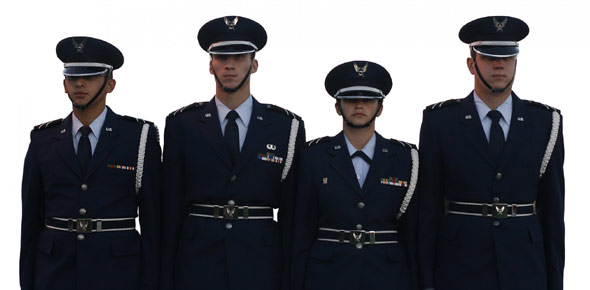# 2A652 CDC's (Pretest 1) 100 Question

31 Questions | Attempts: 702SettingsAerospace Ground Equipment CDC's

• 1.
The effect of noise depends on the
• A.

Deflection, frequency, and volume.

• B.

Frequency, volume, and duration of exposure.

• C.

Deflection, volume, and duration of exposure.

• D.

Deflection, frequency, and duration of exposure.

• 2.
How many valence electrons are needed to make a good conductor?
• A.

3

• B.

4

• C.

5

• D.

6

• 3.
After shutdown a motor continues turning; this is known as
• A.

Inertia

• B.

Impedance

• C.

Inductance

• D.

Eddy currents

• 4.
What component on the New Generation Heater activates the heater control unit(HCU)?
• A.

Heater control switch

• B.

Burner control switch

• C.

Thermostatic sensor

• D.

Oil pressure switch

• 5.
Diagonal cutting pliers are designed to cut
• A.

Bolts

• B.

Rivets

• C.

Small wire

• D.

Hard steel

• 6.
“The flow or drift of electrons through a conductor” in the same direction at the same time is the definition of
• A.

Conductivity

• B.

Voltage

• C.

Current

• D.

Valence

• 7.
The first step of troubleshooting heating equipment is
• A.

Isolation

• B.

Analysis

• C.

Verification

• D.

Recognition

• 8.
Given a voltage of 24 volts and a resistance of 18 ohms in a series circuit, the current through the resistor would be
• A.

.075 amps

• B.

1.33 amps

• C.

133 amps

• D.

750 amps

• 9.
The stator windings of a synchronous motor are wound
• A.

20 degrees apart

• B.

30 degrees apart

• C.

90 degrees apart

• D.

120 degrees apart

• 10.
“Dielectric” is the term used for
• A.

Conductors

• B.

Insulators

• C.

Doped atoms

• D.

Semi-conductors

• 11.
Torque is defined as
• A.

Horsepower

• B.

Wattage

• C.

Rotational force

• D.

Opposition to applied voltage

• 12.
The area around a magnet where its influence can be felt is the definition of the
• A.

Saturation principle

• B.

Magnetic theory

• C.

Magnetic field

• D.

Law of attraction and repulsion

• 13.
The size of a screwdriver is determined by the shank
• A.

Length

• B.

Weight

• C.

Temper

• D.

Width

• 14.
If you need to find out how to perform any maintenance required during an inspection, you should refer to the
• A.

-1 TO

• B.

PE workcards

• C.

Methods and procedures TO

• D.

Service inspection workcards

• 15.
What component on the MA-3D air conditioner allows the operator to control the overall volume of air leaving the unit?
• A.

Blower control switch

• B.

Airflow control switch

• C.

Evaporator control switch

• D.

Temperature control switch

• 16.
Which component is one of the major causes of failure in the DC motor?
• A.

Commutator

• B.

Slip Ring

• C.

Bearings

• D.

Rotor

• 17.
Which part of the torque wrench scale is normally not used?
• A.

Lower 10%

• B.

Lower 20%

• C.

Upper 20%

• D.

Upper 10%

• 18.
The primary function of a nut is to
• A.

Prevent Vibration

• B.

• C.

Provide an auxiliary locking or safety device

• D.

• 19.
When you check the concentricity of a commutator or slips rings, look for
• A.

Wear

• B.

Pitting

• C.

Roundness

• D.

Overheating

• 20.
In a parallel circuit, the total voltage is equal to the
• A.

Sum of the voltage across each branch

• B.

Voltage across each resistor

• C.

Voltage across each branch

• D.

The reciprocal of the voltage across the resistances

• 21.
Heat added to a gaseous substance is known as
• A.

Super heat

• B.

Latent heat

• C.

Specific heat

• D.

Sensible heat

• 22.
Replacement hardware decisions are based on
• A.

Supervisor's preference

• B.

Serviceability criteria

• C.

Availability

• D.

Cost

• 23.
What is the total current in a series-parallel circuit that has a total voltage or 48 volts, a series current of 6 amps, one branch current of 2A, and the other branch resistance of 3 ohms?
• A.

3A

• B.

6A

• C.

8A

• D.

12A

• 24.
The magnetism that remains after the magnetizing force has been removed is known as
• A.

Electromagnetism

• B.

Molecular magnetism

• C.

Permeability

• D.

Residual magnetism

• 25.
If you must push a wrench rather than pull it, how should you do it?
• A.

Grasp the handle near the socket

• B.

Grip the wrench tightly near the center

• C.

Grip the wrench tightly with both hands

• D.Back to top